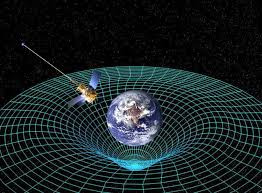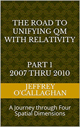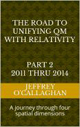# The direction of the mind.

In Neil deGrasse Tyson book Death by Black Hole: And Other Cosmic Quandaries (Kindle Edition Locations 258-262) he tells us "the homing of our senses from birth through childhood allows us, as adults, to pass judgment on events and phenomena in our lives, declaring whether they “make sense”. Problem is, hardly any scientific discoveries of the past century flowed from the direct application of our five senses.  They flowed instead from the direct application of sense-transcendent mathematics and hardware.  This simple fact is entirely responsible for why, to the average person, relativity, particle physics, and 10-dimensional string theory make no sense.

However I disagree with his statement that only a direct application of "sense-transcendent mathematics and hardware" is the only way for us to make sense of the world around us because one advantage human beings have over them is that we can change our perspective on what our senses are telling us whereas they cannot.

For example an artist can "direct the mind" to perceive three-dimensional objects on a two dimensional canvas by using techniques that give one the perspective of three-dimension space.Similarly scientists can and should direct the mind of the "average person" to see the four-dimensional aspects of Relativity by using techniques that would allow them to perceive them in our three-dimensional universe.

For example in Einstein’s General Theory of Relativity he derived gravity in terms of a curvature in the geometry of four dimensional space-time which one can connect to the "real world" by showing how that a planet to revolve around the sun for the same reason a marble revolves around a curvature in a rubber diaphragm.

However he was unable to do the same for electrical forces as was documented by the American Institute of Physics even though he felt "that electromagnetism and gravity could both be explained as aspects of some broader mathematical structure" .

“From before 1920 until his death in 1955, Einstein struggled to find laws of physics far more general than any known before  In his theory of relativity, the force of gravity had become an expression of the geometry of space and time  The other forces in nature, above all the force of electromagnetism, had not been described in such terms  But it seemed likely to Einstein that electromagnetism and gravity could both be explained as aspects of some broader mathematical structure  The quest for such an explanation â€” for a “unified field” theory that would unite electromagnetism and gravity, space and time, all together â€” occupied more of Einstein”s years than any other activity.

One reason he could not define a common mathematical structure for electromagnetism and gravity may have been because human beings do not have the ability to perceive or sense the physical properties of time or a time dimension.  However they can perceive the physical properties of three-dimensional space.

In other words he may have been able to make the connection between gravity and electromagnetism by mathematically viewing their associated forces in terms of how our senses perceive the properties of three-dimensional space.

Einstein gave us the ability to do this when he used the velocity of light and the equation E=mc^2 to define relativistic properties of space-time because it allows one to convert a unit of time in his four dimensional space-time universe to a unit of space in a one consisting of only four *spatial* dimensions.  Additionally because the velocity of light is constant it is possible to defined a one to one correspondence between his space-time universe and one made up of four *spatial* dimensions.

In other words by mathematically defining the relativistic properties of a space-time universe in terms of the constant velocity of light he provided a qualitative and quantitative means of redefining those properties in terms of the geometry of four *spatial* dimensions thereby giving one the ability to define electrical forces in terms our sense perception of the multi directional properties of the spatial dimensions while maintaining those relativistic properties.

The fact that one can use Einstein’s equations to qualitatively and quantitatively redefine the curvature in space-time he associated with gravity in terms of four *spatial* dimensions is one bases for assuming, as was done in the article “Defining energy?” Nov 27, 2007 that all forms of energy including gravitational and electromagnetism can be derived in terms of a spatial displacement in a “surface” of a three-dimensional space manifold with respect to a fourth *spatial* dimension.

This would have allowed him to direct his and the mind of the "average person" to define electrical force in terms of our real world perception of a wave by extrapolating that perception to the movement of a wave in an environment consisting of four spatial dimensions.

For example, a wave on the two-dimensional surface of water causes a point on that surface to be become displaced or rise above or below the equilibrium point that existed before the wave was present.  A force will be developed by the differential displacement of the surfaces, which will result in the elevated and depressed portions of the water moving towards or become "attracted" to each other and the surface of the water.

Similarly, as was shown in the article "What is electromagnetism?" Sept, 27 2007 an electromagnetic wave on the "surface" of a three-dimensional space manifold with respect to a fourth *spatial* dimension would cause a point on that "surface" to become displaced or rise above and below the equilibrium point that existed before the wave was present.

Therefore, our sensory perceptions of three-dimensional space, if extrapolated to four *spatial* dimensions tells us a force will be developed by the differential displacements caused by a matter wave moving on a "surface" of three-dimensional space with respect to a fourth *spatial* dimension that will result in its elevated and depressed portions moving towards or become "attracted" to each other.

This would allow the "average person" to understand the causality of the attractive forces of unlike charges associated with the electromagnetism in terms of a force developed by a differential displacement of a point on a "surface" of a three-dimensional space manifold with respect to a fourth *spatial* dimension.

However, it also provides a mechanism for understanding why similar charges repel each other because observations of water show that there is a direct relationship between the magnitudes of a displacement in its surface to the magnitude of the force resisting that displacement

Similarly the magnitude of a displacement in a "surface" of a three-dimensional space manifold with respect to a fourth *spatial* dimension caused by two similar charges will be greater than that caused by a single one.  Therefore, similar charges will repel each other because the magnitude of the force resisting the displacement will be greater for two charges than it would be for a single charge.

One can define the causality of electrical component of electromagnetic radiation in terms of the energy associated with its "peaks" and "troughs" that is directed perpendicular to its velocity vector while its magnetic component would be associated with the horizontal force developed by that perpendicular displacement.

However, our sensory perception of three-dimensional space also tells us a horizontal force will be developed by that perpendicular or vertical displacement which will always be 90 degrees out of phase with it  This force is called magnetism.

This is analogous to how the vertical force pushing up of on mountain also generates a horizontal force, which pulls matter horizontally towards the apex of that displacement.

This shows how one can explain electrical forces by extrapolating our perception of a three-dimensional environment to one consisting of four *spatial* dimensions and would have allowed him to mathematically integrate them into his space-time model of the universe because, as was shown above they are mathematically identical.

However another advantage to viewing the relativistic properties of our universe in terms of its spatial instead of its time component is that it would allow one to integrate the quantum mechanical properties of electromagnetic energy into the mathematics of General Relativity in a manner that is consistent with our sense perceptions of three-dimensional space.

For example the article “Why is energy/mass quantized?” Oct  4, 2007 showed one can derive the quantized wave properties of electromagnetism by extrapolating our understanding of a resonant structure and perception of a wave in a three-dimensional environment to a electromagnetic wave on a "surface" of a three-dimensional space manifold with respect to a fourth *spatial* dimension.

Briefly it showed the four conditions required for resonance to occur in a three-dimensional environment, an object, or substance with a natural frequency, a forcing function at the same frequency as the natural frequency, the lack of a damping frequency and the ability for the substance to oscillate spatial would occur in one consisting of four spatial dimensions.

The existence of four *spatial* dimensions would give an electromagnetic wave the ability to oscillate spatially on a "surface" between a third and fourth *spatial* dimensions thereby fulfilling one of the requirements for classical resonance to occur.

These oscillations would be caused by an event such as the decay of a subatomic particle or the shifting of an electron in an atomic orbital.  This would force the "surface" of a three-dimensional space manifold to oscillate with the frequency associated with the energy of that event.

The oscillations caused by such an event would serve as forcing function allowing a resonant system or "structure" to be established space.

Therefore, these oscillations in a "surface" of a three-dimensional space manifold would meet the requirements mentioned above for the formation of a resonant system or "structure" in four-dimensional space if one extrapolated them to that environment.

In our three-dimensional environment the energy of a resonant system can only take on the discrete or quantized values associated with it fundamental or a harmonic of its fundamental frequency.

Hence, these resonant systems in four *spatial* dimensions would be responsible for the discrete quantized energy associated with the quantum mechanical properties of a photon or an electromagnetic field.

Yet one can also define its boundary conditions of its resonate structure in the terms of our perceptions of a three dimensional environment.

For example in our three-dimensional world, a point on the two-dimensional surface of paper is confined to that surface.  However, that surface can oscillate up or down with respect to three-dimensional space.

Similarly an object occupying a volume of three-dimensional space would be confined to it however, it could, similar to the surface of the paper oscillate “up” or “down” with respect to a fourth *spatial* dimension.

The confinement of the “upward” and “downward” oscillations of an electromagnetic wave with respect to a fourth *spatial* dimension is what defines the spatial boundaries associated with a particle in the article â€œWhy is energy/mass quantized?" Oct 4, 2007.

Additionally one of the most advantageous results of viewing the relativistic properties of Einstein’s theories in terms of their spatial instead of its time components is that it allow for the integration of one of most perplexing aspects of quantum mechanics; that of how and why a particle position when observed is based only on probabilities.

The physics of wave mechanics tell us that due to their continuous properties the energy waves the article "Why is energy/mass quantized?" Oct. 4, 2007 associated with a quantum system would be distributed throughout the entire "surface" a three-dimensional space manifold with respect to a fourth *spatial* dimension.

For example the energy of a vibrating or oscillating ball on a rubber diaphragm would be disturbed over its entire surface while the magnitude of those vibrations would decrease as one move away from the focal point of the oscillations.

Similarly if the assumption that quantum properties of energy are a result of vibrations or oscillations in a "surface" of three-dimensional space is correct those oscillations would be distributed over the entire "surface" three-dimensional space while the magnitude of those vibrations would be greatest at the focal point of the oscillations and decreases as one moves away from it.

As mentioned earlier the article “Why is energy/mass quantized?” shown a quantum particle is a result of a resonant structure formed on the "surface" of a three-dimensional space manifold with respect to a fourth *spatial* dimension.

Yet the science of Wave Mechanics tells us resonance would most probably occur on the surface of the rubber sheet were the magnitude of the vibrations is greatest and would diminish as one move away from that point,

Similarly a particle would most probably be found were the magnitude of the vibrations in a "surface" of a three-dimensional space manifold is greatest and would diminish as one move away from that point.

This shows how one can physically connect the probabilities associated quantum mechanics to our observable environment by redefining them in terms of the relativistic properties of Einstein’s space-time universe in terms of four *spatial* dimensions.

In other words by changing our perspective on fundamental make up of our universe from one of quantized parts to the relativistic properties of four *spatial* dimensions or four dimensional space time may allow us to ingrate the world of quantum mechanics into the space-time-universe of gravity defined by Einstein because as was shown above his mathematics tell us they are interchangeable.

Additionally as mentioned earlier Einstein felt "that electromagnetism and gravity could both be explained as aspects of some broader mathematical structure".

The above discussion vindicates that belief because it shows that one can not only incorporate gravity and electromagnetism but also its quantum properties into a broader mathematical structure by rewriting the mathematics of space-time in terms of the relativistic properties of four *spatial* dimensions.

It also shows the advantages of "directing the mind" to perceive how three-dimensional space would interact with four by using techniques such as those outlined above to understand how they are responsible for the reality we all see around us.

It should be remember that Einstein’s genius allows us to choose whether to create physical images of an unseen "reality" in either a space-time environment or one consisting of four *spatial* dimension when he defined the geometry of space-time in terms of the constant velocity of light.

Later Jeff

 The Road to Unifying QM with Relativity part 1 2007 thru 2010Ebook \$8.00Paper Back \$15.00 The Road to Unifying QM with Relativity part 2 2011 thru 2014Ebook \$8.00Paper Back \$16.00 The Road to Unifying QM with Relativity part 3 2015 thru 2020Ebook \$8.00Paper Back \$18.00